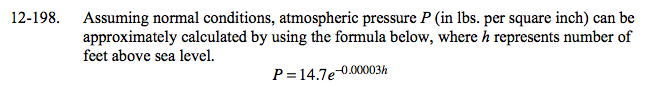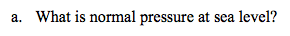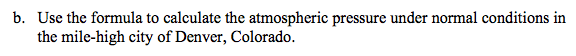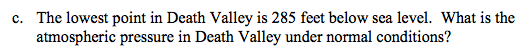### Home > A2C > Chapter 12 > Lesson 12.5.1 > Problem12-198

12-198.
1. Assuming normal conditions, atmospheric pressure P (in lbs. per square inch) can be approximately calculated by using the formula below, where h represents number of feet above sea level. Homework Help ✎

2. P = 14.7e−0.00003h

1. What is normal pressure at sea level?

2. Use the formula to calculate the atmospheric pressure under normal conditions in the mile-high city of Denver, Colorado.

3. The lowest point in Death Valley is 285 feet below sea level. What is the atmospheric pressure in Death Valley under normal conditions?P = 14.7e−0.00003(0)

14.7 PsiP = 14.7e−0.00003(5280)P = 14.7e−0.00003(−285)